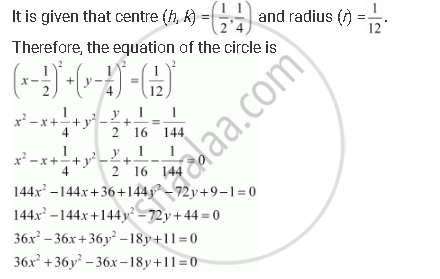CBSE (Arts) Class 11CBSE
Share
Notifications

View all notifications
Books Shortlist
Your shortlist is empty

# Find the Equation of the Circle with (1/2, 1/4)And Radius 1/12 - CBSE (Arts) Class 11 - Mathematics

Login
Create free account

Forgot password?

#### Question

Find the equation of the circle with (1/2, 1/4)and radius 1/12

#### Solution

The equation of a circle with centre (hk) and radius is given as

(x­ – h)2 + (y ­– k)2 = r2Is there an error in this question or solution?

#### APPEARS IN

Solution Find the Equation of the Circle with (1/2, 1/4)And Radius 1/12 Concept: Concept of Circle.
S